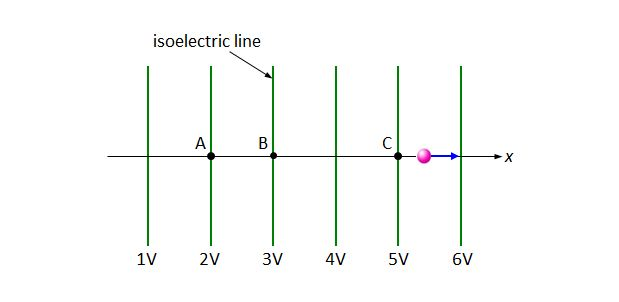# Accelerating in electric fieldA point charge is in uniformly accelerated motion (in the $+x$ direction) in a uniform electric field. After being put at point A, it passes through points B and C, as shown above. Let $v_B$ and $v_C$ denote the speeds of the point charge at points B and C, respectively. Then what is the ratio $v_B:v_C?$

×